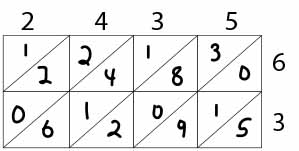# Lattice Method of Multiplication

Instructions:

question 1 of 3

### What's the product (answer)? Complete the lattice table.Create Your Account To Take This Quiz

As a member, you'll also get unlimited access to over 79,000 lessons in math, English, science, history, and more. Plus, get practice tests, quizzes, and personalized coaching to help you succeed.

Try it risk-free for 30 days. Cancel anytime

### 2. What is a lattice?

Create your account to access this entire worksheet
Quizzes, practice exams & worksheets
Certificate of Completion
Create an account to get started

The questions in this quiz/worksheet are designed to test how well you understand lattice multiplication by breaking down its components and giving you the chance to tackle an example problem.

## Quiz & Worksheet Goals

This quiz/worksheet includes questions involving:

• Completing a lattice table
• The steps of lattice multiplication
• Defining lattice
• The history of the lattice method
• What the lattice method is similar to in mathematics

## Skills Practiced

• Problem solving - use acquired knowledge to solve a problem by working with a lattice table
• Defining key concepts - ensure that you can accurately define lattice
• Information recall - access the knowledge you've gained regarding how old the lattice method is as well as what to do after multiplying the numbers in a lattice table
• Distinguishing differences - make sure you can differentiate between mathematical tables that are and aren't similar to the lattice method

• Familiarize yourself with how to set up a lattice table
• See how the lattice method is performed within a provided example
• Know what the lattice method is named for
Final ExamMath for Kids
Status: Not Started
Chapter ExamMath Basics for Elementary School
Status: Not Started

Support